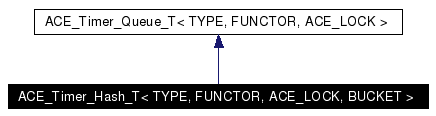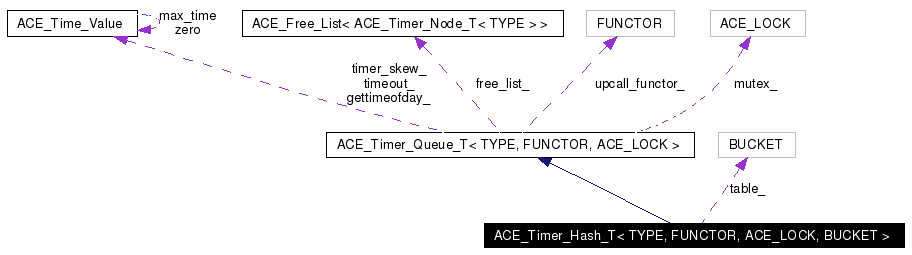# ACE_Timer_Hash_T< TYPE, FUNCTOR, ACE_LOCK, BUCKET > Class Template Reference

Provides a hash table of <bucket>s as an implementation for a timer queue. More...

`#include <Timer_Hash_T.h>`

Inheritance diagram for ACE_Timer_Hash_T< TYPE, FUNCTOR, ACE_LOCK, BUCKET >:[legend]
Collaboration diagram for ACE_Timer_Hash_T< TYPE, FUNCTOR, ACE_LOCK, BUCKET >:[legend]
List of all members.

## Public Types

typedef ACE_Timer_Hash_Iterator_T<
TYPE, FUNCTOR, ACE_LOCK,
BUCKET >
HASH_ITERATOR
Type of iterator.
typedef ACE_Timer_Queue_T<
TYPE, FUNCTOR, ACE_LOCK >
INHERITED
Type inherited from.

## Public Member Functions

ACE_Timer_Hash_T (size_t table_size, FUNCTOR *upcall_functor=0, ACE_Free_List< ACE_Timer_Node_T< TYPE > > *freelist=0)
ACE_Timer_Hash_T (FUNCTOR *upcall_functor=0, ACE_Free_List< ACE_Timer_Node_T< TYPE > > *freelist=0)
virtual ~ACE_Timer_Hash_T (void)
Destructor.
virtual int is_empty (void) const
True if queue is empty, else false.
virtual const ACE_Time_Valueearliest_time (void) const
virtual int reset_interval (long timer_id, const ACE_Time_Value &interval)
virtual int cancel (const TYPE &type, int dont_call_handle_close=1)
virtual int cancel (long timer_id, const void **act=0, int dont_call_handle_close=1)
virtual int expire (void)
virtual int expire (const ACE_Time_Value &current_time)
virtual ACE_Timer_Queue_Iterator_T<
TYPE, FUNCTOR, ACE_LOCK > &
iter (void)
Returns a pointer to this <ace_timer_queue>'s iterator.
virtual ACE_Timer_Node_T<
TYPE > *
remove_first (void)
Removes the earliest node from the queue and returns it.
virtual void dump (void) const
Dump the state of an object.
virtual ACE_Timer_Node_T<
TYPE > *
get_first (void)
Reads the earliest node from the queue and returns it.

## Private Member Functions

virtual long schedule_i (const TYPE &type, const void *act, const ACE_Time_Value &future_time, const ACE_Time_Value &interval)
virtual int dispatch_info_i (const ACE_Time_Value &current_time, ACE_Timer_Node_Dispatch_Info_T< TYPE > &info)
Non-locking version of dispatch_info ().
virtual void reschedule (ACE_Timer_Node_T< TYPE > *)
Reschedule an "interval" <ace_timer_node>.
void find_new_earliest (void)
Finds the earliest node.
ACE_Timer_Hash_T (const ACE_Timer_Hash_T< TYPE, FUNCTOR, ACE_LOCK, BUCKET > &)
void operator= (const ACE_Timer_Hash_T< TYPE, FUNCTOR, ACE_LOCK, BUCKET > &)

## Private Attributes

size_t size_
Keeps track of the size of the queue.
BUCKET ** table_
Table of BUCKETS.
size_t table_size_
Keeps track of the size of table_.
ACE_Timer_Hash_Upcall< TYPE,
FUNCTOR, ACE_LOCK >
table_functor_
Functor used for the table's timer queues.
size_t earliest_position_
Index to the position with the earliest entry.
HASH_ITERATORiterator_
Iterator used to expire timers.

## Friends

class ACE_Timer_Hash_Iterator_T<TYPE, FUNCTOR, ACE_LOCK, BUCKET>
Iterator is a friend.

## Detailed Description

### template<class TYPE, class FUNCTOR, class ACE_LOCK, class BUCKET> class ACE_Timer_Hash_T< TYPE, FUNCTOR, ACE_LOCK, BUCKET >

Provides a hash table of <bucket>s as an implementation for a timer queue.

This implementation uses a hash table of BUCKETs. The hash is based on the time_value of the event. Unlike other Timer Queues, ACE_Timer_Hash does not expire events in order.

## Member Typedef Documentation

 template typedef ACE_Timer_Hash_Iterator_T ACE_Timer_Hash_T< TYPE, FUNCTOR, ACE_LOCK, BUCKET >::HASH_ITERATOR
 Type of iterator.

 template typedef ACE_Timer_Queue_T ACE_Timer_Hash_T< TYPE, FUNCTOR, ACE_LOCK, BUCKET >::INHERITED
 Type inherited from.

## Constructor & Destructor Documentation

 template ACE_Timer_Hash_T< TYPE, FUNCTOR, ACE_LOCK, BUCKET >::ACE_Timer_Hash_T ( size_t table_size, FUNCTOR * upcall_functor = `0`, ACE_Free_List< ACE_Timer_Node_T< TYPE > > * freelist = `0` )
 Default constructor. determines the size of the hash table. is the instance of the FUNCTOR to be used by the buckets. If is 0, a default FUNCTOR will be created.

 template ACE_Timer_Hash_T< TYPE, FUNCTOR, ACE_LOCK, BUCKET >::ACE_Timer_Hash_T ( FUNCTOR * upcall_functor = `0`, ACE_Free_List< ACE_Timer_Node_T< TYPE > > * freelist = `0` )
 Default constructor. is the instance of the FUNCTOR to be used by the queue. If is 0, Timer Hash will create a default FUNCTOR. the freelist of timer nodes. If 0, then a default freelist will be created. The default size will be ACE_DEFAULT_TIMERS and there will be no preallocation.

 template ACE_Timer_Hash_T< TYPE, FUNCTOR, ACE_LOCK, BUCKET >::~ACE_Timer_Hash_T ( void ) ` [virtual]`
 Destructor.

 template ACE_Timer_Hash_T< TYPE, FUNCTOR, ACE_LOCK, BUCKET >::ACE_Timer_Hash_T ( const ACE_Timer_Hash_T< TYPE, FUNCTOR, ACE_LOCK, BUCKET > & ) ` [private]`

## Member Function Documentation

 template int ACE_Timer_Hash_T< TYPE, FUNCTOR, ACE_LOCK, BUCKET >::cancel ( long timer_id, const void ** act = `0`, int dont_call_handle_close = `1` ) ` [virtual]`
 Cancel the single timer that matches the value (which was returned from the method). If act is non-NULL then it will be set to point to the ``magic cookie'' argument passed in when the timer was registered. This makes it possible to free up the memory and avoid memory leaks. If is 0 then the will be invoked. Returns 1 if cancellation succeeded and 0 if the wasn't found. Implements ACE_Timer_Queue_T< TYPE, FUNCTOR, ACE_LOCK >.

 template int ACE_Timer_Hash_T< TYPE, FUNCTOR, ACE_LOCK, BUCKET >::cancel ( const TYPE & type, int dont_call_handle_close = `1` ) ` [virtual]`
 Cancel all timer associated with . If is 0 then the will be invoked. Returns number of timers cancelled. Implements ACE_Timer_Queue_T< TYPE, FUNCTOR, ACE_LOCK >.

 template int ACE_Timer_Hash_T< TYPE, FUNCTOR, ACE_LOCK, BUCKET >::dispatch_info_i ( const ACE_Time_Value & current_time, ACE_Timer_Node_Dispatch_Info_T< TYPE > & info ) ` [private, virtual]`
 Non-locking version of dispatch_info (). Reimplemented from ACE_Timer_Queue_T< TYPE, FUNCTOR, ACE_LOCK >.

 template void ACE_Timer_Hash_T< TYPE, FUNCTOR, ACE_LOCK, BUCKET >::dump ( void ) const` [virtual]`
 Dump the state of an object. Reimplemented from ACE_Timer_Queue_T< TYPE, FUNCTOR, ACE_LOCK >.

 template const ACE_Time_Value & ACE_Timer_Hash_T< TYPE, FUNCTOR, ACE_LOCK, BUCKET >::earliest_time ( void ) const` [virtual]`
 Returns the time of the earlier node in the . Must be called on a non-empty queue. Implements ACE_Timer_Queue_T< TYPE, FUNCTOR, ACE_LOCK >.

 template int ACE_Timer_Hash_T< TYPE, FUNCTOR, ACE_LOCK, BUCKET >::expire ( const ACE_Time_Value & current_time ) ` [virtual]`
 Run the for all timers whose values are <= . This does not account for . Returns the number of timers canceled. Reimplemented from ACE_Timer_Queue_T< TYPE, FUNCTOR, ACE_LOCK >.

 template int ACE_Timer_Hash_T< TYPE, FUNCTOR, ACE_LOCK, BUCKET >::expire ( void ) ` [virtual]`
 Run the for all timers whose values are <= . Also accounts for . Returns the number of timers canceled. Reimplemented from ACE_Timer_Queue_T< TYPE, FUNCTOR, ACE_LOCK >.

 template void ACE_Timer_Hash_T< TYPE, FUNCTOR, ACE_LOCK, BUCKET >::find_new_earliest ( void ) ` [private]`
 Finds the earliest node.

 template ACE_Timer_Node_T< TYPE > * ACE_Timer_Hash_T< TYPE, FUNCTOR, ACE_LOCK, BUCKET >::get_first ( void ) ` [virtual]`
 Reads the earliest node from the queue and returns it. Implements ACE_Timer_Queue_T< TYPE, FUNCTOR, ACE_LOCK >.

 template int ACE_Timer_Hash_T< TYPE, FUNCTOR, ACE_LOCK, BUCKET >::is_empty ( void ) const` [virtual]`
 True if queue is empty, else false. Implements ACE_Timer_Queue_T< TYPE, FUNCTOR, ACE_LOCK >.

 template ACE_Timer_Queue_Iterator_T< TYPE, FUNCTOR, ACE_LOCK > & ACE_Timer_Hash_T< TYPE, FUNCTOR, ACE_LOCK, BUCKET >::iter ( void ) ` [virtual]`
 Returns a pointer to this 's iterator. Implements ACE_Timer_Queue_T< TYPE, FUNCTOR, ACE_LOCK >.

 template void ACE_Timer_Hash_T< TYPE, FUNCTOR, ACE_LOCK, BUCKET >::operator= ( const ACE_Timer_Hash_T< TYPE, FUNCTOR, ACE_LOCK, BUCKET > & ) ` [private]`

 template ACE_Timer_Node_T< TYPE > * ACE_Timer_Hash_T< TYPE, FUNCTOR, ACE_LOCK, BUCKET >::remove_first ( void ) ` [virtual]`
 Removes the earliest node from the queue and returns it. Implements ACE_Timer_Queue_T< TYPE, FUNCTOR, ACE_LOCK >.

 template void ACE_Timer_Hash_T< TYPE, FUNCTOR, ACE_LOCK, BUCKET >::reschedule ( ACE_Timer_Node_T< TYPE > * ) ` [private, virtual]`
 Reschedule an "interval" . Implements ACE_Timer_Queue_T< TYPE, FUNCTOR, ACE_LOCK >.

 template int ACE_Timer_Hash_T< TYPE, FUNCTOR, ACE_LOCK, BUCKET >::reset_interval ( long timer_id, const ACE_Time_Value & interval ) ` [virtual]`
 Resets the interval of the timer represented by to , which is specified in relative time to the current . If is equal to , the timer will become a non-rescheduling timer. Returns 0 if successful, -1 if not. Implements ACE_Timer_Queue_T< TYPE, FUNCTOR, ACE_LOCK >.

 template long ACE_Timer_Hash_T< TYPE, FUNCTOR, ACE_LOCK, BUCKET >::schedule_i ( const TYPE & type, const void * act, const ACE_Time_Value & future_time, const ACE_Time_Value & interval ) ` [private, virtual]`
 Schedule that will expire at , which is specified in absolute time. If it expires then is passed in as the value to the . If is != to then it is used to reschedule the automatically, using relative time to the current . This method returns a that is a pointer to a token which stores information about the event. This can be used to cancel the timer before it expires. Returns -1 on failure. Implements ACE_Timer_Queue_T< TYPE, FUNCTOR, ACE_LOCK >.

## Friends And Related Function Documentation

 template friend class ACE_Timer_Hash_Iterator_T` [friend]`
 Iterator is a friend.

## Member Data Documentation

 template size_t ACE_Timer_Hash_T< TYPE, FUNCTOR, ACE_LOCK, BUCKET >::earliest_position_` [private]`
 Index to the position with the earliest entry.

 template HASH_ITERATOR* ACE_Timer_Hash_T< TYPE, FUNCTOR, ACE_LOCK, BUCKET >::iterator_` [private]`
 Iterator used to expire timers.

 template size_t ACE_Timer_Hash_T< TYPE, FUNCTOR, ACE_LOCK, BUCKET >::size_` [private]`
 Keeps track of the size of the queue.

 template BUCKET** ACE_Timer_Hash_T< TYPE, FUNCTOR, ACE_LOCK, BUCKET >::table_` [private]`
 Table of BUCKETS.

 template ACE_Timer_Hash_Upcall ACE_Timer_Hash_T< TYPE, FUNCTOR, ACE_LOCK, BUCKET >::table_functor_` [private]`
 Functor used for the table's timer queues.

 template size_t ACE_Timer_Hash_T< TYPE, FUNCTOR, ACE_LOCK, BUCKET >::table_size_` [private]`
 Keeps track of the size of table_.

The documentation for this class was generated from the following files:
Generated on Thu Feb 10 20:36:49 2005 for ACE by1.3.9.1